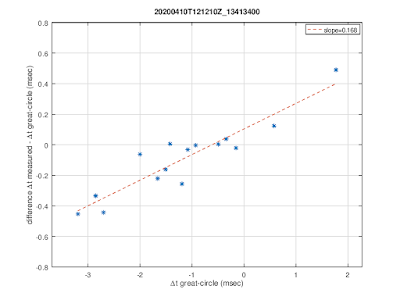## Friday, April 10, 2020

### HF TDoA multilateration (1)

This blog post contains an analysis of TDoA multilateration applied to signal on 13413.4 kHz using a number of KiwiSDRs located in Europe.

For now the assumption used for making the KiwiSDR TDoA maps is that signals propagate with speed of light along the ground. Here we compare the measured delay differences with the ones obtained from this assumption.

All plots shown in this blog post are generated using octave/matlab .mat files available when using the updated KiwiSDR TDoA algorithm.

The cross-correlations for all combinations of used KiwiSDRs, normalized to have their maximum at unity, are shown below.cross-correlations

Differences between the measured values and the ones obtained from great-circle-derived time delay differences are due to ionospheric propagation. As expected, the ionospheric effects tend to cancel for pairs of KiwiSDRs which are at about the same distance to the transmitter:Comparison of  measured with great-circle time differences

The following scatter plot shows the effect of ionospheric propagation w.r.t. great-circle propagation. It will be very interesting to re-do this analysis using propagation delays e.g. from VOACAP instead of assuming propagation along great-circles at ground level.Scatter plot for time differences

Slightly earlier the plots looked like this:cross-correlationsComparison of  measured with great-circle time differencesScatter plot for time differences